# Python 交易回測工具 Backtesting.py 簡易教學

· 7 分鐘閱讀1. 首先要能取得股價：請參考【如何使用Python取得歷史股價，簡介yfinance、ffn、FinMind
2. 要回測技術指標，當然要能產生取得技術指標：請參考【如何使用Python產生技術指標？TA-Lib簡易教學

## 簡介 Backtesting.py​

pip3 install backtesting

### 官網 sample code 說明​

from backtesting import Backtest, Strategyfrom backtesting.lib import crossoverfrom backtesting.test import SMA, GOOGclass SmaCross(Strategy):    n1 = 10    n2 = 20    def init(self):        close = self.data.Close        self.sma1 = self.I(SMA, close, self.n1)        self.sma2 = self.I(SMA, close, self.n2)    def next(self):        if crossover(self.sma1, self.sma2):            self.buy()        elif crossover(self.sma2, self.sma1):            self.sell()bt = Backtest(GOOG, SmaCross,              cash=10000, commission=.002,              exclusive_orders=True)output = bt.run()bt.plot()

• 從 backtesting.test 取得 GOOG 的股價
• 從 backtesting.test 使用 SMA(移動平均) function 取得 10 日和 20 日的移動平均值
• 從 backtesting.lib 使用 crossover function 執行策略：
當 10 日移動平均往上穿越 20 日移動平均時「買進」
當 10 日移動平均往下穿越 20 日移動平均時「賣出」
• 範例的三個參數：
cash: 初始的現金
commission: 交易成本，就交易一次的手續費、交易稅的總合
exclusive_orders: True 代表每筆新交易會關閉上一次的交易

print output 可以看到以下的資料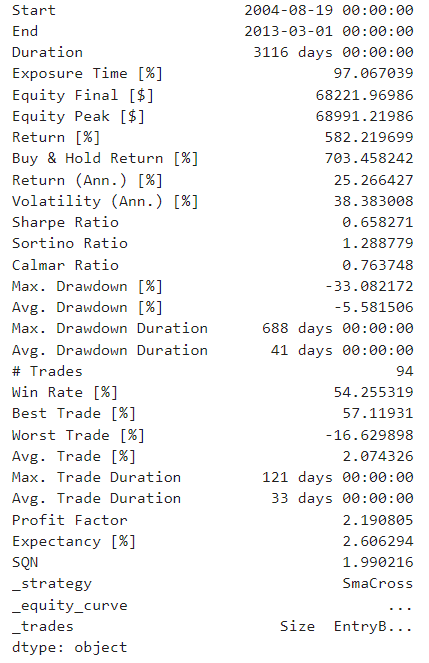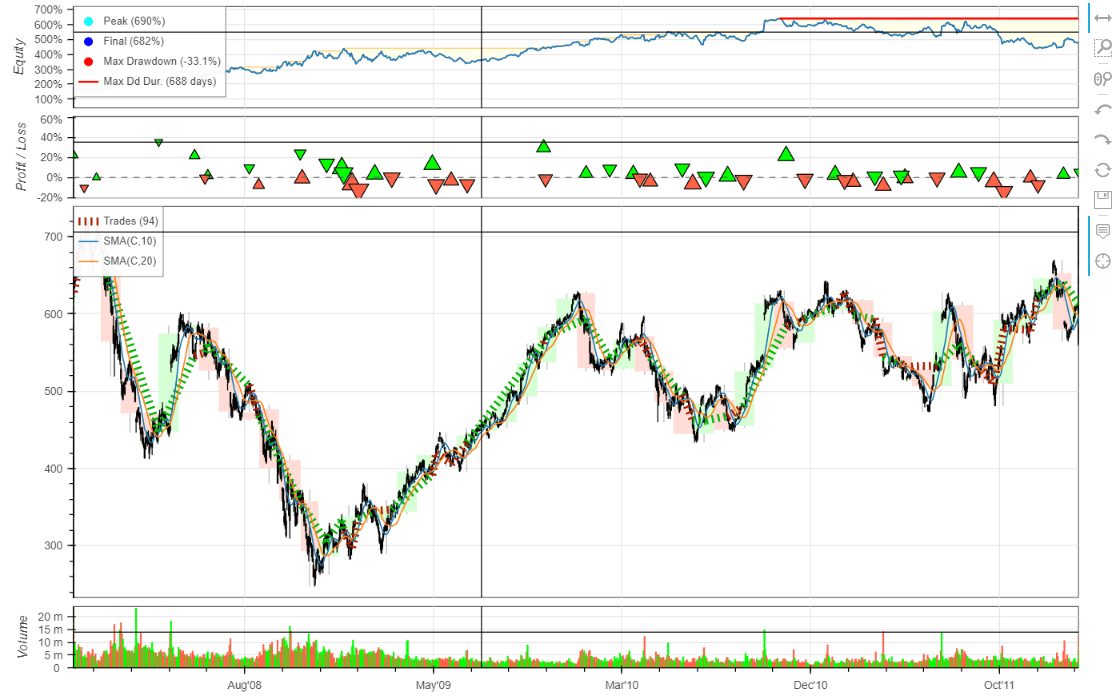## 置換資料源​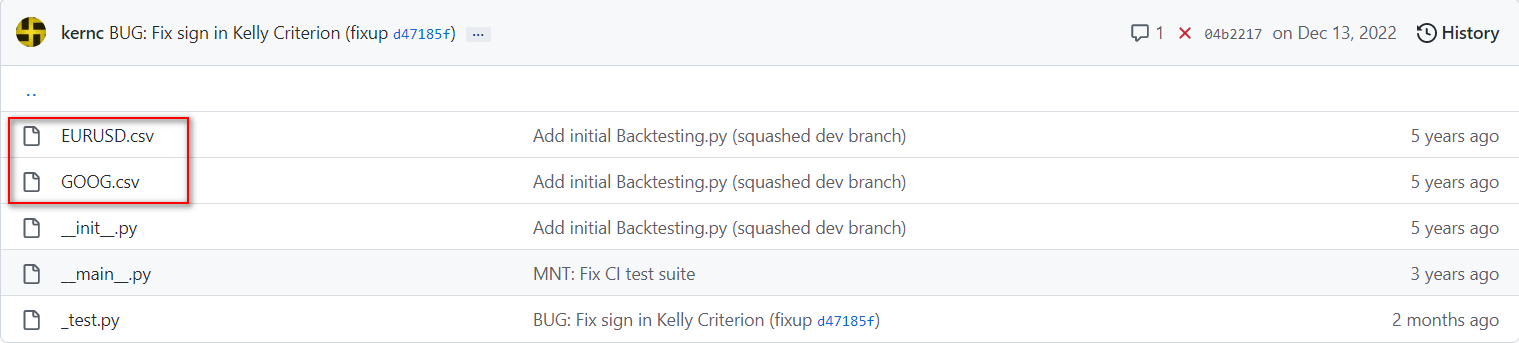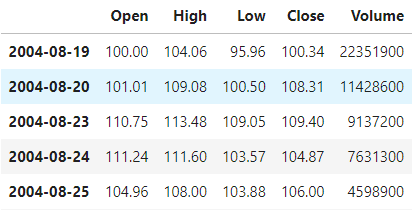FinMind 的資料就要做些轉換，像是將欄位第一個字母改成大寫 ex: open => Open，以及將 index 改為日期的型態，完整程式如下

from FinMind.data import DataLoaderimport pandas as pddl = DataLoader()df = dl.taiwan_stock_daily(stock_id='0050', start_date='2003-01-01', end_date='2023-02-25')df = df.rename(columns={"date": "Date"})df.set_index("Date" , inplace=True)df = df.rename(columns={"open": "Open", "max": "High", "min": "Low", "close": "Close", "Trading_Volume": "Volume"})df = df.rename(columns={"Trading_Volume": "Volume"})df = df.set_index(pd.DatetimeIndex(pd.to_datetime(df.index)))

## 置換技術指標​

sample code 中有 SMA(移動平均)，好像也有技術指標了？實際上看一下 Github 原始碼，可以看到它其實就只有一個 SMA functoin，沒別的了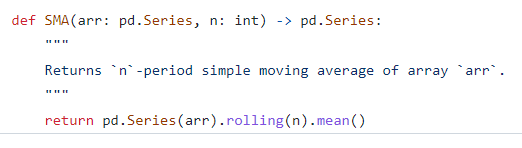from backtesting import Backtest, Strategyfrom backtesting.lib import crossoverfrom FinMind.data import DataLoaderimport pandas as pdimport talibfrom talib import abstract## 取得資料dl = DataLoader()df = dl.taiwan_stock_daily(stock_id='0050', start_date='2003-01-01', end_date='2023-02-25')## 整理資料格式df = df.rename(columns={"date": "Date"})df.set_index("Date" , inplace=True)df = df.set_index(pd.DatetimeIndex(pd.to_datetime(df.index)))## backtesting.py 格式df1 = df.rename(columns={"open": "Open", "max": "High", "min": "Low", "close": "Close", "Trading_Volume": "Volume"})## ta-lib 格式df2 = df.rename(columns={"max": "high", "min": "low", "Trading_Volume": "Volume"})## 取得 KD 值df_kd = abstract.STOCH(df2,fastk_period=9, slowk_period=3,slowd_period=3)## 合併資料df1 = pd.merge(df1, df_kd, on="Date")## KD 策略class KdCross(Strategy):    def init(self):        super().init()            def next(self):        if crossover(20, self.data.slowk): ## K<20 買進            self.buy()        elif crossover(self.data.slowk, 80): ## K>80 平倉            self.position.close()            bt = Backtest(df1, KdCross, cash=10000, commission=.001798) ## 交易成本 0.1798%output = bt.run()bt.plot()